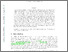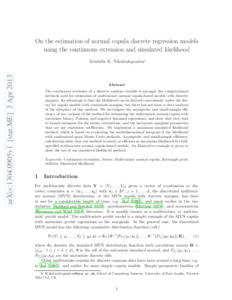# On the estimation of normal copula discrete regression models using the continuous extension and simulated likelihood

Nikoloulopoulos, Aristidis K.ORCID: https://orcid.org/0000-0003-0853-0084 (2013) On the estimation of normal copula discrete regression models using the continuous extension and simulated likelihood. Journal of Statistical Planning and Inference, 143 (11). pp. 1923-1937. ISSN 0378-3758Preview PDF (1304.0905v1) - Accepted Version Download (266kB) | Preview

## Abstract

The continuous extension of a discrete random variable is amongst the computational methods used for estimation of multivariate normal copula-based models with discrete margins. Its advantage is that the likelihood can be derived conveniently under the theory for copula models with continuous margins, but there has not been a clear analysis of the adequacy of this method. We investigate the asymptotic and small-sample efficiency of two variants of the method for estimating the multivariate normal copula with univariate binary, Poisson, and negative binomial regressions, and show that they lead to biased estimates for the latent correlations, and the univariate marginal parameters that are not regression coefficients. We implement a maximum simulated likelihood method, which is based on evaluating the multidimensional integrals of the likelihood with randomized quasi-Monte Carlo methods. Asymptotic and small-sample efficiency calculations show that our method is nearly as efficient as maximum likelihood for fully specified multivariate normal copula-based models. An illustrative example is given to show the use of our simulated likelihood method.

Item Type: Article continuous extension,jitters,multivariate normal copula,rectangle probabilities,simulated likelihood Faculty of Science > School of Computing Sciences Faculty of Science > Research Groups > Data Science and StatisticsFaculty of Science > Research Groups > Statistics http://arxiv.org/abs/1304.0905 Pure Connector 28 Sep 2013 00:47 05 Dec 2023 01:49 https://ueaeprints.uea.ac.uk/id/eprint/43513 10.1016/j.jspi.2013.06.015View Item